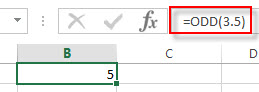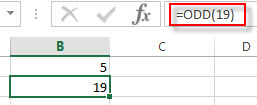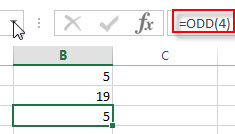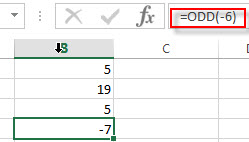# Excel ODD Function

This post will guide you how to use Excel ODD function with syntax and examples in Microsoft excel.

### Description

The Excel ODD function rounds a given number up to the nearest odd integer. So you can use the ODD function to return the next ODD integer after rounding a supplied number up. The returned value is away from zero. It means that if the number is a positive value and the return value will become larger and if the number is a negative value, the return value will become smaller.

The ODD function is a build-in function in Microsoft Excel and it is categorized as a Math and Trigonometry Function.

The ODD function in Excel 2016, Excel 2013, Excel 2010, Excel 2007, Excel 2003, Excel XP, Excel 2000, Excel 2011 for Mac.

### Syntax

The syntax of the ODD function is as below:

`=ODD (number)`

Where the ODD function arguments is:

• number -This is a required argument. . A numeric value that you want to round up to the nearest ODD integer.

Note:

• If the number argument is not numeric value, the ODD function will return the #VALUE! Error.
• If the number is an odd integer, the ODD function will do not any rounding.

### Excel ODD Function Examples

The below examples will show you how to use Excel ODD Function to round up a given number to the nearest even integer.

1# to round 3.5 up to the nearest ODD integer, enter the following formula in Cell B1.

`=ODD(3.5)`2# to round 19 to the nearest ODD integer, enter the following formula in Cell B2.

`=ODD (19)`3# to round 4 up to the nearest ODD integer, enter the following formula in Cell B3.

`=ODD (4)`4# to round -6 up to the nearest ODD integer, enter the following formula in Cell B4.

`=ODD (-6)`### Related Functions

• Excel EVEN function
The Excel EVEN function rounds a given number up to the nearest even integer. So you can use the EVEN function to return the next even integer after rounding a supplied number up.The syntax of the EVEN function is as below:=EVEN (number)…
• Excel ROUND function
The Excel ROUND function rounds a number to a specified number of digits. You can use the ROUND function to round to the left or right of the decimal point in Excel.The syntax of the ROUND function is as below:=ROUND (number, num_digits)…
• Excel ROUNDDOWN function
The Excel ROUNDDOWN function round the number down to the specified number of digits. The syntax of the ROUNDDOWN function is as below:=ROUNDDOWN (number, num_digits)…
• Excel ROUNDUP function
The Excel ROUNDUP function rounds the number up to a specified number of decimal places. It will round away from 0.The syntax of the ROUNDUP function is as below:=ROUNDUP (number, num_digits)…
• Excel CEILING Function
The Excel CEILING function returns a given number rounded up to the nearest multiple of a given number of significance. The syntax of the CEILING function is as below:= CEILING (number, significance)…
• Excel CEILING.PRECISE Function
The Excel CEILING.PRECISE function returns a given number rounded up to the nearest multiple of a given number of significance or returns a number that is rounded up to the nearest integer number.The syntax of the CEILING.PRECISE function is as below:=CEILING.PRECISE (number, [significance])…
Related Posts

Excel DVAR Function

This post will guide you how to use Excel DVAR function with syntax and examples in Microsoft excel. Description The Excel DVAR Function will get the variance of a population based on a sample of numbers in a column in ...

Excel DSUM Function

This post will guide you how to use Excel DSUM function with syntax and examples in Microsoft excel. Description The Excel DSUM Function will add the numbers in a column or database that meets a given criteria. And so you ...

Excel DSTDEVP Function

This post will guide you how to use Excel DSTDEVP function with syntax and examples in Microsoft excel. Description The Excel DSTDEVP returns the standard deviation of a population based on the entire population of numbers in a column or ...

Excel DSTDEV Function

This post will guide you how to use Excel DSTDEV function with syntax and examples in Microsoft excel. Description The Excel DSTDEV returns the standard deviation of a population based on a sample of numbers in a column or database ...

Excel DMIN Function

This post will guide you how to use Excel DMIN function with syntax and examples in Microsoft excel. Description The Excel DMIN returns the minimum value from a database or a column of a list that matches the specified conditions. ...

Excel DPRODUCT Function

This post will guide you how to use Excel DPRODUCT function with syntax and examples in Microsoft excel. Description The Excel DPRODUCT returns the product of values from a set of records that match criteria that you specify. The DPRODUCT ...

Excel XIRR Function

This post will guide you how to use Excel XIRR function with syntax and examples in Microsoft excel. Description The Excel XIRR function returns the internal rate of return for a series of cash flows that is not necessarily periodic. ...

Excel VDB Function

This post will guide you how to use Excel VDB function with syntax and examples in Microsoft excel. Description The Excel VDB function calculates the depreciation of an asset for a specified period based on the double declining balance method. ...

Excel SYD Function

This post will guide you how to use Excel SYD function with syntax and examples in Microsoft excel. Description The Excel SYD function calculates the sum-of-years’digits depreciation of an asset for a specified period. And it will return a numeric ...

Excel SLN Function

This post will guide you how to use Excel SLN function with syntax and examples in Microsoft excel. Description The Excel SLN function calculates the depreciation of an asset for one period based on the straight line depreciation. And it ...

Sidebar